# Highlights

• Simple off-policy algorithm that can match or surpass SoTA RL algorithms
• Can be used with a static dataset with no modifications

# Introduction

Model-free reinforcement learning can be a general and effective methodology for training agents to acquire sophisticated behaviors with minimal assumptions on the underlying task. However, reinforcement learning algorithms can be substantially more complex to implement and tune than standard supervised learning methods. [The goal of this paper is to present] a reinforcement learning algorithm that is simple, easy to implement, and can readily incorporate off-policy experience data.

Advantage-Weighted Regression (AWR) is a simple off-policy algorithm for model-free RL. Each iteration of the AWR algorithm simply consists of two supervised regression steps: one for training a value function baseline via regression onto cumulative rewards, and another for training the policy via weighted regression.

# Background

Let’s remember the basic RL objective: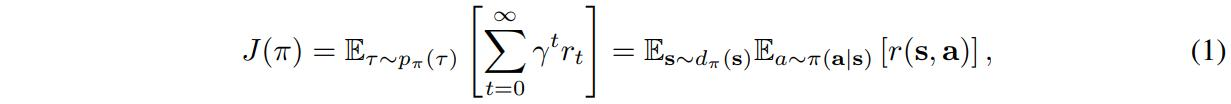AWR builds on reward-weighted regression (RWR), a policy search algorithm based on an expectation-maximization framework, which solves at each iteration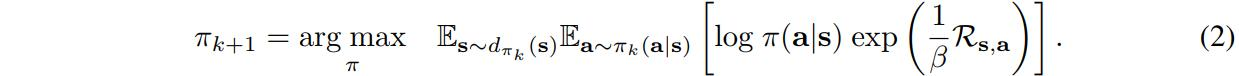which can be interpreted as solving a maximum likelihood problem that fits a new policy $$\pi_{k+1}$$ to samples collected under the current policy $$\pi_{k}$$

# Method

The major improvement that AWR provides is adding a baseline to the policy update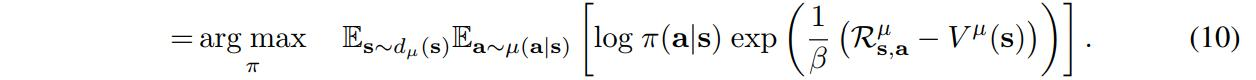Following a set of complicated derivations, the authors also extend the algorithm so the samples can come not only from the last policy but from a mixture of policies from past iterations.

The algorithm then becomes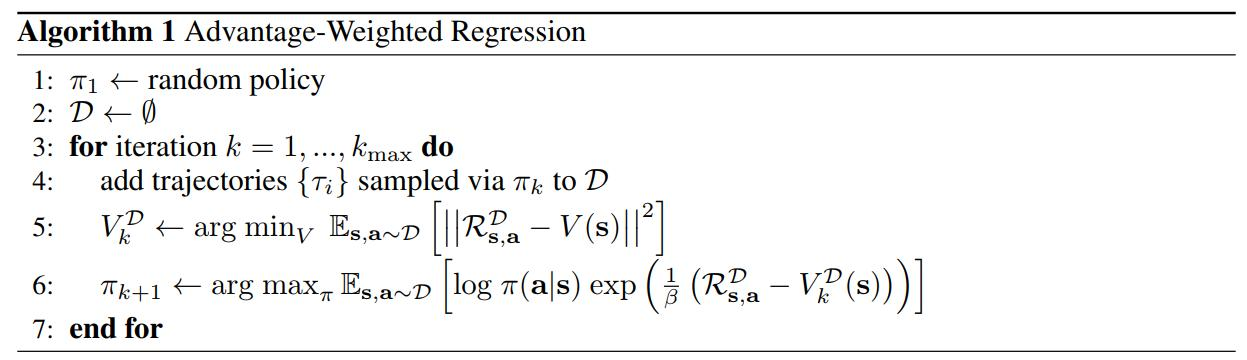where $$V(s)$$ is defined via $$TD(\lambda)$$ learning. Because the algorithm is off-policy, $$D$$ can also be a static dataset of trajectories.

## Data

Experiments were performed on several OpenAI Gym tasks.

# Results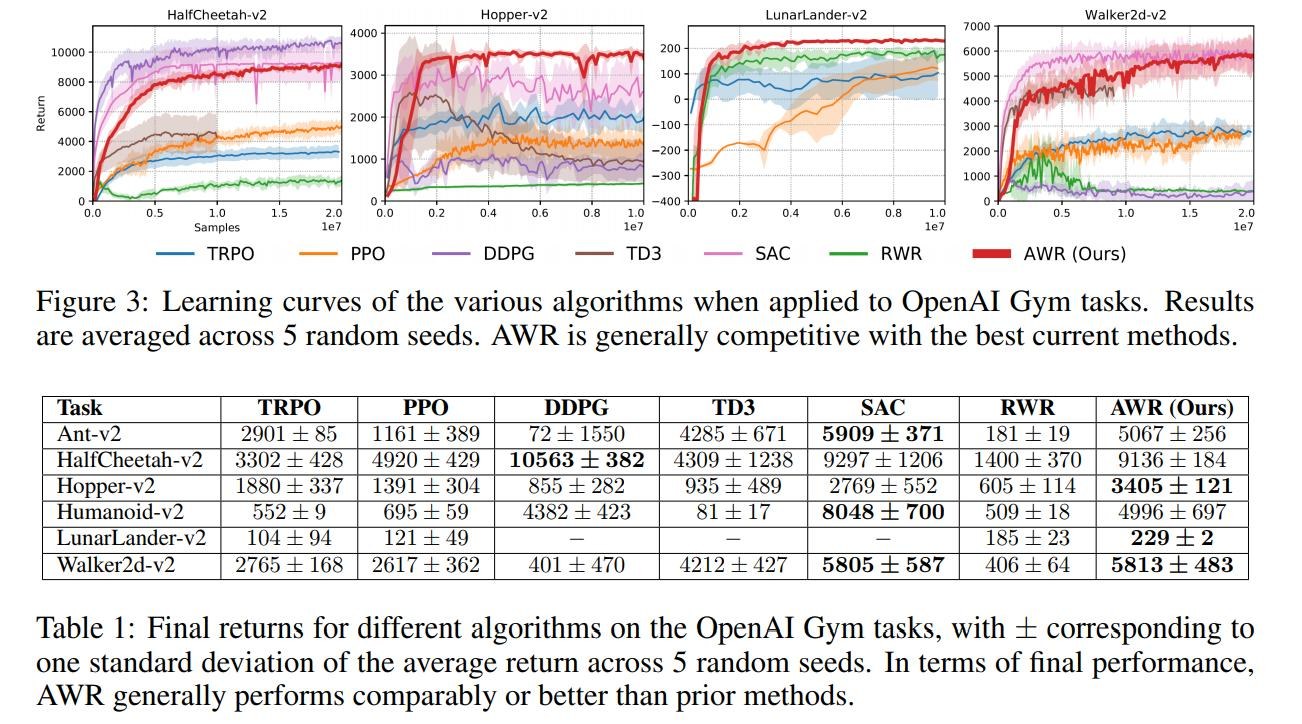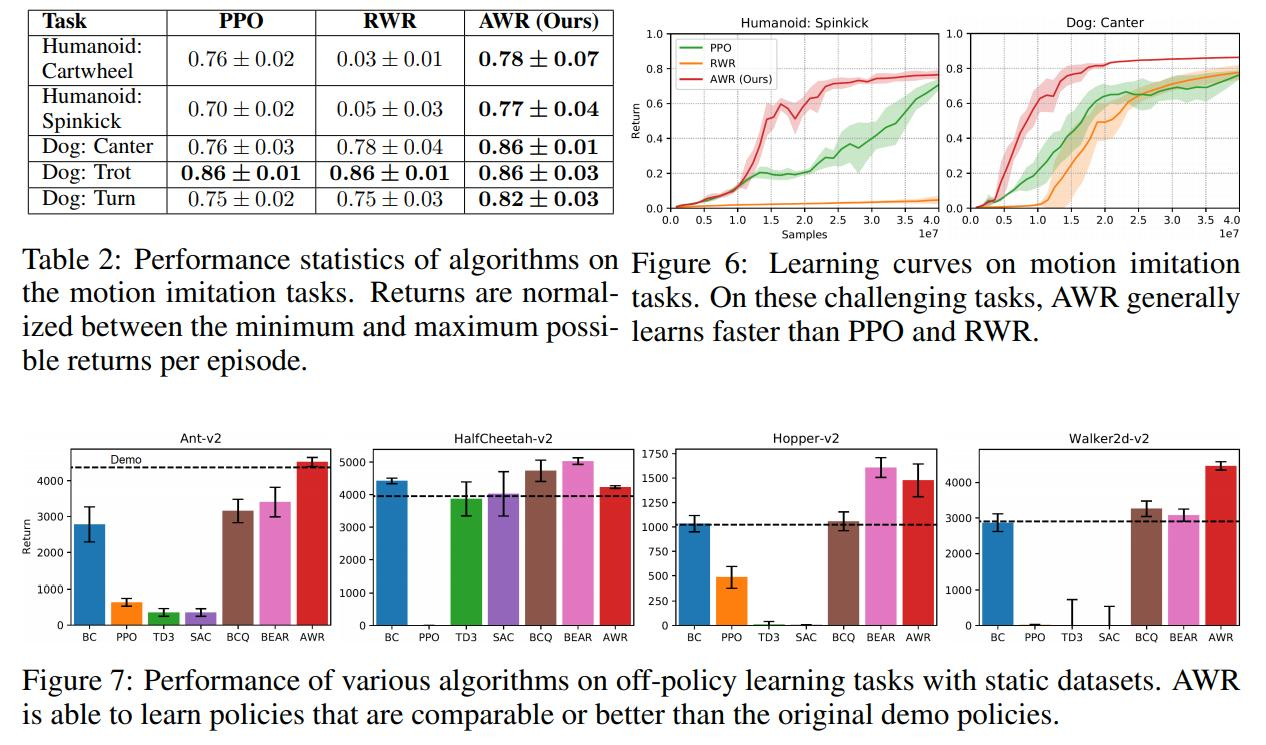# Conclusions

Despite its simplicity, [AWR] is able to solve challenging control tasks with complex simulated agents, and achieve competitive performance on standard benchmarks compared to a number of well-established RL algorithms. AWR is also able to learn from fully off-policy datasets, demonstrating comparable performance to state-of-the-art off-policy methods.

Landing page

Code

Video